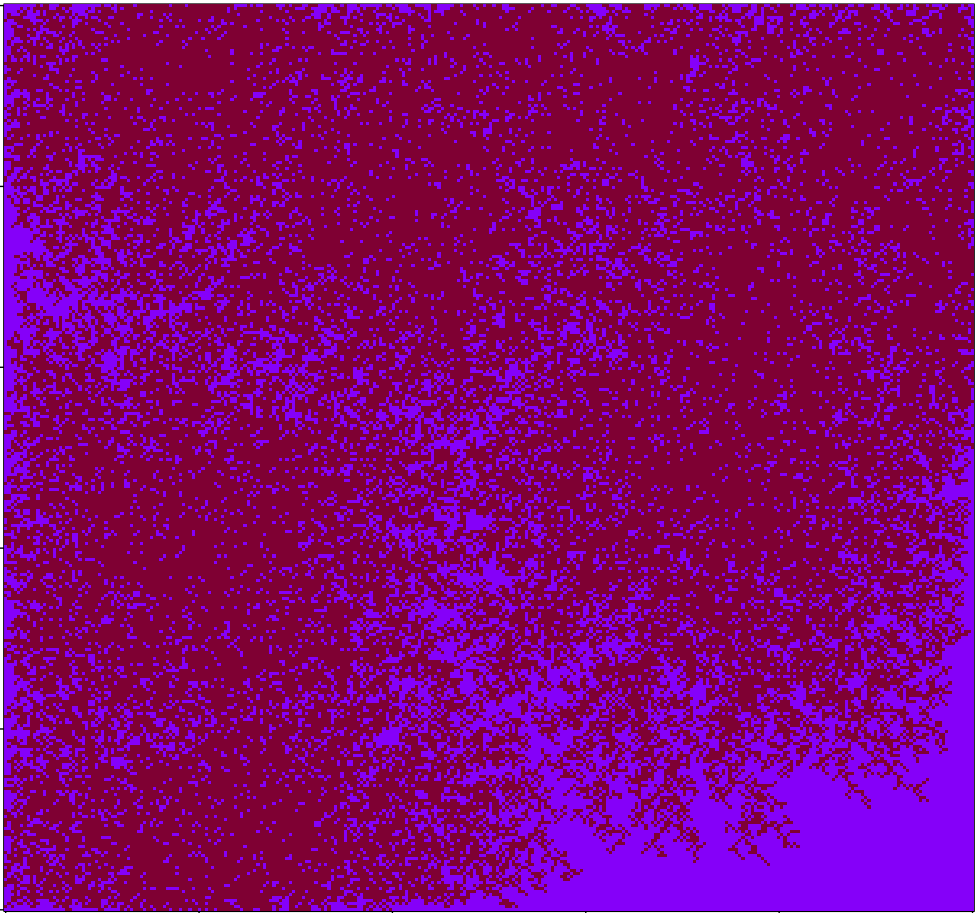## Population simulation leads to Valentine’s Day a[R]tWorking on a quick-and-dirty simulation of people wandering around until they find neighbors, then settling down. After playing with the coloring a bit I arrived at the above image, which I quite like. Code below:

```# Code by Matt Asher for statisticsblog.com
# Feel free to modify and redistribute, but please keep this notice

maxSettlers = 150000

# Size of the area
areaW = 300
areaH = 300

# How many small movements will they make to find a neighbor
maxSteps = 200

areaMatrix = matrix(0, nrow=areaW, ncol=areaH)

# For the walk part

# Is an adjacent cell occupied?
hasNeighbor <- function(m,n,theMatrix) {
toReturn = FALSE
for(k in 1:8) {
if( !((xCheck > areaW) | (xCheck < 1) | (yCheck > areaH) | (yCheck < 1)) ) {
if(theMatrix[yCheck,xCheck]>0) {
toReturn = TRUE
}
}
}
return(toReturn)
}

# Main loop
for(i in 1:maxSettlers) {
steps = 1
xPos = sample(1:areaW, 1)
yPos = sample(1:areaH, 1)

areaMatrix[xPos,yPos] = 1
} else {
if(areaMatrix[xPos,yPos]==0 & hasNeighbor(xPos,yPos,areaMatrix)) {
areaMatrix[xPos,yPos] = 1
} else {
spotFound = FALSE
outOfBounds = FALSE

while(!spotFound & !outOfBounds & (steps areaW) | (xPos < 1) | (yPos > areaH) | (yPos < 1)) {
outOfBounds = TRUE
} else if(hasNeighbor(xPos,yPos,areaMatrix) ) {
areaMatrix[xPos,yPos] = steps
spotFound = TRUE
}
}
}

}

}

image(areaMatrix, col=rev(rgb(seq(0.01,1,0.01),seq(0.01,1,0.01),seq(0.01,1,0.01))))

# I think this version looks nicer!
# areaMatrix[areaMatrix !=0] = 1
# image(areaMatrix, col=rev(rgb(.5,0,seq(0.2,1,0.2))))
```

Tags:

1.Richard
2.Jan Henzl